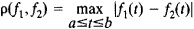Function Space

(redirected from Space of function)

function space

[′fəŋk·shən ‚spās]
(mathematics)
A metric space whose elements are functions.

Function Space

a set of functions for which there is defined a notion of distance or, more generally, of proximity between any two functions. A function space is called linear if, along with any two elements f1 and f2 it contains all their linear combinations αf1, + βf2, where α and β are real or complex numbers. An example of a linear function space is the space C(a, b) of all continuous functions on the interval [a, b] with the distance p(f1, f2) between two functions being given by the formulaFunction spaces are the most important concrete linear spaces studied in functional analysis. [28–388–1 ]

References in periodicals archive ?
is a Hilbert Space of function analytic in the unit disk with the inner product [<f,g>.
This volume examines the Gaussian random fields using the structure of the Hilbert space of functions on the parameter space associated with the covariance, or the Reproducing Kernel Hilbert Space (RKHS), with applications of these ideas to research in finance, spatial statistics, and the filtering and analytic problem related to fractional Brownian motion.
p] space of functions f defined on R endowed with the following finite norm
The first main result of this paper proves embeddings of certain modulation spaces into VMO, the space of functions with vanishing mean oscillation.
Gautam's results  make clear that membership in VMO (the space of functions of vanishing mean oscillation) is central to the Balian-Low Theorem.
For a choice of appropriate weights we can identify the space of functions with certain smoothness and decay properties as a modulation space.
For this reason, one can construct a Hilbert space of functions that can be used for describing the given system as an eigenfunction of the Hamiltonian.
i] is assumed to be in some predefined space of functions [H.

Site: Follow: Share:
Open / Close